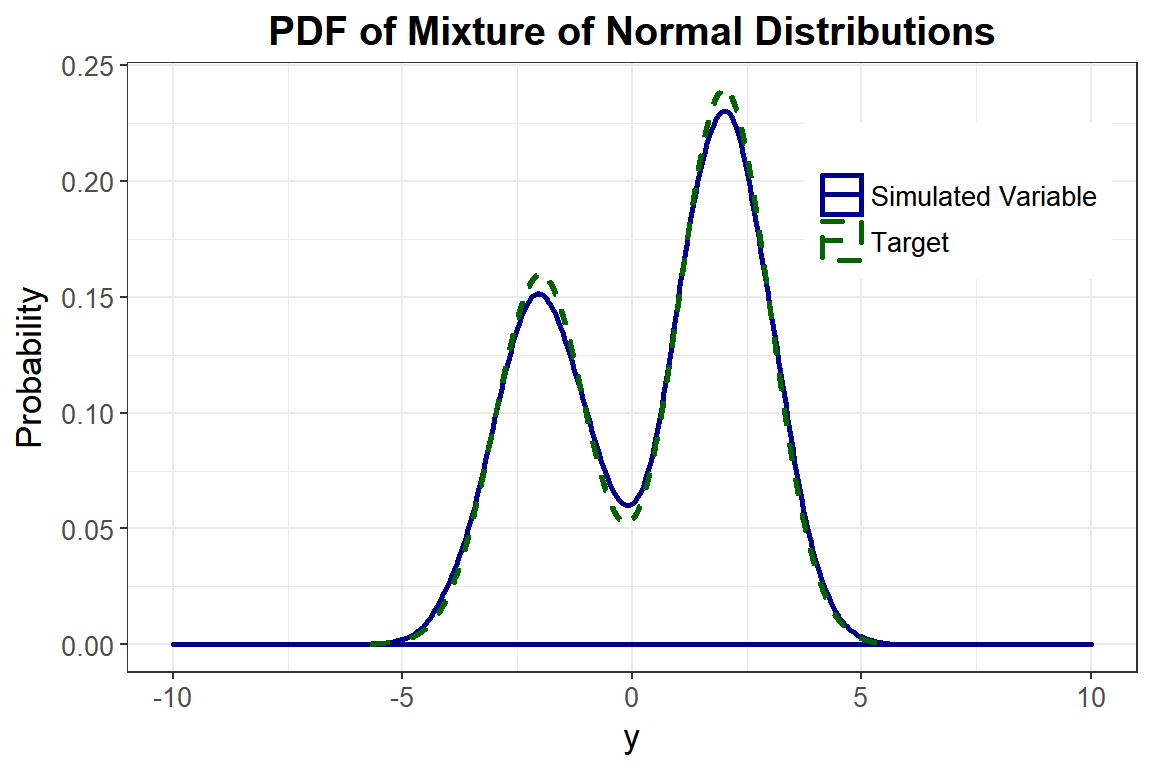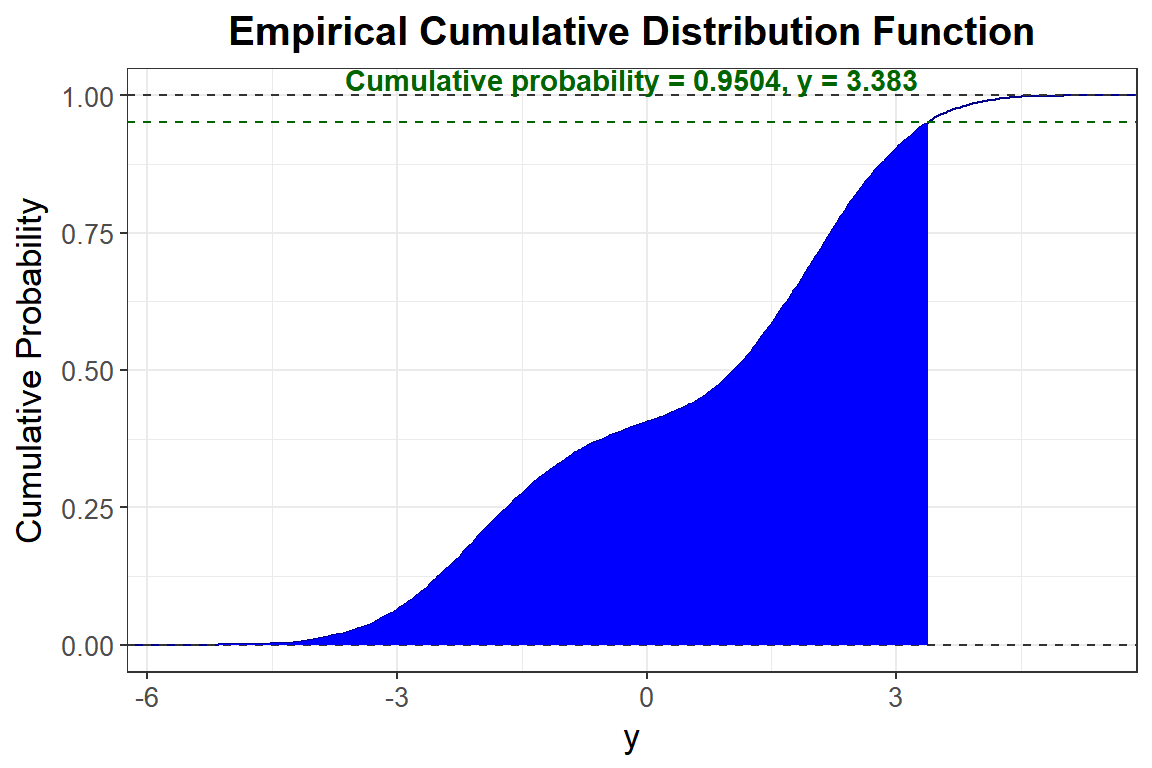The following example demonstrates the use of the contmixvar1 function, which simulates one continuous mixture distribution $$Y$$. Headrick (2002)’s fifth-order transformation is used to generate the components of $$Y$$ with n = 10000. Headrick and Kowalchuk (2007) outlined a general method for comparing a simulated distribution $$Y$$ to a given theoretical distribution $$Y^*$$. These have been modified below to apply to continuous mixture variables and will be shown for Example 1. Note that they could easily be modified further for comparison to an empirical vector of data. More details about continuous mixture variables can be found in the Expected Cumulants and Correlations for Continuous Mixture Variables vignette. Some code has been modified from the SimMultiCorrData package (Fialkowski 2018).

1. Obtain the standardized cumulants (skewness, kurtosis, fifth, and sixth) for the components of $$Y^*$$. This can be done using SimMultiCorrData::calc_theory along with either the component distribution names (plus up to 4 parameters for each) or the PDF’s with support bounds. In the case of an empirical vector of data, use SimMultiCorrData::calc_moments or SimMultiCorrData::calc_fisherk (Fialkowski 2018). If you desire $$Y$$ to have the mean and variance of $$Y^*$$, use calc_mixmoments with the component parameters as inputs to find $$E[Y^*]$$ and $$SD[Y^*]$$.

2. Obtain the constants for the components of $$Y$$. This can be done using SimMultiCorrData::find_constants on each component or by simulating the mixture variable with contmixvar1.

3. Determine whether these constants produce a valid power method PDF. The results of find_constants or contmixvar1 indicate whether the constants yield invalid or valid PDF’s for the component variables. The constants may also be checked using SimMultiCorrData::pdf_check. If the constants generate an invalid PDF, the user should check if the skurtosis falls above the lower bound (using SimMultiCorrData::calc_lower_skurt). If yes, sixth cumulant correction values should be used in SimMultiCorrData::find_constants or contmixvar1 to find the smallest corrections that produce valid PDF constants. If all of the component distributions have valid PDF’s, the mixture variable has a valid PDF.

4. Select a critical value from $$Y^*$$, i.e. $$y^*$$ such that $$Pr(Y^* \ge y^*) = \alpha$$ for the desired significance level $$\alpha$$.

5. Calculate the cumulative probability for the simulated mixture variable $$Y$$ up to $$y^*$$ and compare to $$1 - \alpha$$.

6. Plot a parametric graph of $$Y^*$$ and $$Y$$. This can be done with the simulated vector of data $$Y$$ using plot_simpdf_theory (overlay = TRUE) and specifying the PDF fx for the mixture variable. If comparing to an empirical vector of data, use SimMultiCorrData::plot_sim_pdf_ext.

# Example: Mixture of 2 Normal Distributions

The component distributions are Normal(-2, 1) and Normal(2, 1). The mixing proportions are $$0.4$$ and $$0.6$$. Use Headrick and Kowalchuk (2007)’s steps to compare the density of the simulated variable to the theoretical density:

## Step 1: Obtain the standardized cumulants

The values of $$\gamma_1,\ \gamma_2,\ \gamma_3,$$ and $$\gamma_4$$ are all $$0$$ for normal variables. The mean and standard deviation of the mixture variable are found with calc_mixmoments.

library("SimCorrMix")
## Loading required package: SimMultiCorrData
##
## Attaching package: 'SimMultiCorrData'
## The following object is masked from 'package:stats':
##
##     poly
library("printr")
options(scipen = 999)
n <- 10000
mix_pis <- c(0.4, 0.6)
mix_mus <- c(-2, 2)
mix_sigmas <- c(1, 1)
mix_skews <- rep(0, 2)
mix_skurts <- rep(0, 2)
mix_fifths <- rep(0, 2)
mix_sixths <- rep(0, 2)
Nstcum <- calc_mixmoments(mix_pis, mix_mus, mix_sigmas, mix_skews,
mix_skurts, mix_fifths, mix_sixths)

## Step 2: Simulate the variable

Note that calc_mixmoments returns the standard deviation, not the variance. The simulation functions require variance as the input. First, the parameter inputs are checked with validpar.

validpar(k_mix = 1, method = "Polynomial", means = Nstcum,
vars = Nstcum^2, mix_pis = mix_pis, mix_mus = mix_mus,
mix_sigmas = mix_sigmas, mix_skews = mix_skews, mix_skurts = mix_skurts,
mix_fifths = mix_fifths, mix_sixths = mix_sixths)
##  TRUE
Nmix2 <- contmixvar1(n, "Polynomial", Nstcum, Nstcum^2, mix_pis, mix_mus,
mix_sigmas, mix_skews, mix_skurts, mix_fifths, mix_sixths)
## Total Simulation time: 0.001 minutes

Look at a summary of the target distribution and compare to a summary of the simulated distribution.

SumN <- summary_var(Y_comp = Nmix2$Y_comp, Y_mix = Nmix2$Y_mix,
means = Nstcum, vars = Nstcum^2, mix_pis = mix_pis, mix_mus = mix_mus,
mix_sigmas = mix_sigmas, mix_skews = mix_skews, mix_skurts = mix_skurts,
mix_fifths = mix_fifths, mix_sixths = mix_sixths)
knitr::kable(SumN$target_mix, digits = 5, row.names = FALSE, caption = "Summary of Target Distribution") Table 1: Summary of Target Distribution Distribution Mean SD Skew Skurtosis Fifth Sixth 1 0.4 2.2 -0.2885 -1.15402 1.79302 6.17327 knitr::kable(SumN$mix_sum, digits = 5, row.names = FALSE,
caption = "Summary of Simulated Distribution")
Table 1: Summary of Simulated Distribution
Distribution N Mean SD Median Min Max Skew Skurtosis Fifth Sixth
1 10000 0.4 2.19989 1.05078 -5.69433 5.341 -0.2996 -1.15847 1.84723 6.1398

## Step 3: Determine if the constants generate a valid PDF

Nmix2$constants c0 c1 c2 c3 c4 c5 0 1 0 0 0 0 0 1 0 0 0 0 Nmix2$valid.pdf
##  "TRUE" "TRUE"

## Step 4: Select a critical value

Let $$\alpha = 0.05$$. Since there are no quantile functions for mixture distributions, determine where the cumulative probability equals $$1 - \alpha = 0.95$$. The boundaries for uniroot were determined through trial and error.

fx <- function(x) 0.4 * dnorm(x, -2, 1) + 0.6 * dnorm(x, 2, 1)
cfx <- function(x, alpha, FUN = fx) {
integrate(function(x, FUN = fx) FUN(x), -Inf, x, subdivisions = 1000,
stop.on.error = FALSE)$value - (1 - alpha) } y_star <- uniroot(cfx, c(3.3, 3.4), tol = 0.001, alpha = 0.05)$root
y_star
##  3.382993

## Step 5: Calculate the cumulative probability for the simulated variable up to $$1 - \alpha$$

We will use the function SimMultiCorrData::sim_cdf_prob to determine the cumulative probability for $$Y$$ up to y_star. This function is based on Martin Maechler’s ecdf function (R Core Team 2018).

sim_cdf_prob(sim_y = Nmix2$Y_mix[, 1], delta = y_star)$cumulative_prob
##  0.9504

This is approximately equal to the $$1 - \alpha$$ value of $$0.95$$, indicating the method provides a good approximation to the actual distribution.

## Step 6: Plot graphs

plot_simpdf_theory(sim_y = Nmix2$Y_mix[, 1], ylower = -10, yupper = 10, title = "PDF of Mixture of Normal Distributions", fx = fx, lower = -Inf, upper = Inf)We can also plot the empirical cdf and show the cumulative probability up to y_star. plot_sim_cdf(sim_y = Nmix2$Y_mix[, 1], calc_cprob = TRUE, delta = y_star)